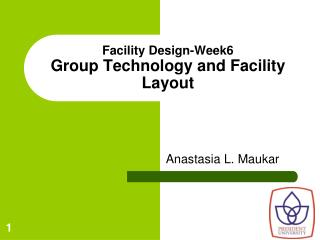DownloadDownload PresentationFacility Design-Week6 Group Technology and Facility Layout

# Facility Design-Week6 Group Technology and Facility Layout

Download Presentation## Facility Design-Week6 Group Technology and Facility Layout

- - - - - - - - - - - - - - - - - - - - - - - - - - - E N D - - - - - - - - - - - - - - - - - - - - - - - - - - -
##### Presentation Transcript

1. Facility Design-Week6Group Technology and Facility Layout Anastasia L. Maukar

2. Introduction Group technology was introduced by Frederick Taylor in 1919 as a way to improve productivity. One of long term benefits of group technology is it helps implement a manufacturing strategy aimed at greater automation.

3. WHAT IS GROUP TECHNOLOGY? Group technology (GT) is a philosophy that impliesthe notion of recognizing and exploiting similarities in three different ways: 1. By performing like activities together 2. By standardizing similar tasks 3. By efficiently storing and retrieving information about recurring problems

4. What is Group Technology Group Technology examines products, parts and assemblies. It then groups similar items to simplify design, manufacturing, purchasing and other business processes.

5. REDUCTIONS Setup time Inventory Material handling cost Direct and indirect labor cost IMPROVEMENTS Quality Material Flow Machine and operator Utilization Space Utilization Employee Morale Benefits of GT and Cellular Manufacturing (CM)

6. Group technology emphasis on part families based on similarities in design attributes and manufacturing, therefore GT contributes to the integration of CAD and CAM.

7. The Basic Key Features for a Successful Group Technology Applications: • Group Layout • Short Cycle Flow Control • A Planned Machine Loading

8. Process layout

9. Group technology layout

10. Sample part-machine processing indicator matrix

11. Rearranged part-machine processing indicator matrix

12. Rearranged part-machine processing indicator matrix

13. Rearranged part-machine processing indicator matrix

14. Classification and Coding Schemes • Hierarchical • Non-hierarchical • Hybrid

15. Classification and Coding Schemes

16. Classification and Coding Schemes

17. Advantages of Classification and Coding Systems • Maximize design efficiency • Maximize process planning efficiency • Simplify scheduling

18. Clustering Approach • Rank order clustering • Bond energy • Row and column masking • Similarity coefficient • Mathematical Programming

19. Rank Order Clustering Algorithm Step 1: Assign binary weight BWj = 2m-j to each column j of the part-machine processing indicator matrix. Step 2: Determine the decimal equivalent DE of the binary value of each row i using the formula Step 3: Rank the rows in decreasing order of their DE values. Break ties arbitrarily. Rearrange the rows based on this ranking. If no rearrangement is necessary, stop; otherwise go to step 4.

20. Rank Order Clustering Algorithm Step 4: For each rearranged row of the matrix, assign binary weight BWi = 2n-i. Step 5: Determine the decimal equivalent of the binary value of each column j using the formula Step 6: Rank the columns in decreasing order of their DE values. Break ties arbitrarily. Rearrange the columns based on this ranking. If no rearrangement is necessary, stop; otherwise go to step 1.

21. Rank Order Clustering – Example 1

22. Rank Order Clustering – Example 1

23. Rank Order Clustering – Example 1

24. Rank Order Clustering – Example 1

25. ROC Algorithm Solution – Example 1

26. Bond Energy Algorithm Step 1: Set i=1. Arbitrarily select any row and place it. Step 2: Place each of the remaining n-i rows in each of the i+1 positions (i.e. above and below the previously placed i rows) and determine the row bond energy for each placement using the formula Select the row that increases the bond energy the most and place it in the corresponding position.

27. Bond Energy Algorithm Step 3: Set i=i+1. If i < n, go to step 2; otherwise go to step 4. Step 4: Set j=1. Arbitrarily select any column and place it. Step 5: Place each of the remaining m-j rows in each of the j+1 positions (i.e. to the left and right of the previously placed j columns) and determine the column bond energy for each placement using the formula Step 6: Set j=j+1. If j < m, go to step 5; otherwise stop.

28. BEA – Example 2

29. BEA – Example 2

30. BEA – Example 2

31. BEA – Example 2

32. BEA Solution – Example 2

33. Row and Column Masking Algorithm Step 1: Draw a horizontal line through the first row. Select any 1 entry in the matrix through which there is only one line. Step 2: If the entry has a horizontal line, go to step 2a. If the entry has a vertical line, go to step 2b. Step 2a: Draw a vertical line through the column in which this 1 entry appears. Go to step 3. Step 2b: Draw a horizontal line through the row in which this 1 entry appears. Go to step 3. Step 3:If there are any 1 entries with only one line through them, select any one and go to step 2. Repeat until there are no such entries left. Identify the corresponding machine cell and part family. Go to step 4. Step 4: Select any row through which there is no line. If there are no such rows, STOP. Otherwise draw a horizontal line through this row, select any 1 entry in the matrix through which there is only one line and go to Step 2.

34. R&CM Algorithm – Example 3

35. R&CM Algorithm – Example 3

36. R&CM Algorithm - Solution

37. Similarity Coefficient (SC) Algorithm

38. SC Algorithm – Example 4

39. SC Algorithm – Example 4

40. SC Algorithm – Example 4

41. SC Algorithm – Example 4

42. SC Algorithm Solution – Example 4